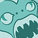Integration, Extension & APIs

Announcements
QlikWorld 2022, LIVE in Denver CO., May 16-19, 2022. REGISTER NOW TO RECEIVE EARLY BIRD PRICING
cancel
Showing results for
Did you mean:Partner

Convert Advance Analytics Tool Box Expression to KPI Expression

HI,

Can any one help me to work below expression in KPI object? Below one is captured from "Console window in Chrome" which was produced from a "Analytics Tool Box extension"

R.ScriptEvalExStr('N', 'save(q,file="~/debug_holt_winters.rda"); library(jsonlite);library(dplyr);library(forecast);data<-ts(na.omit(q\$Measure) ,frequency=12);

res<-forecast(fit, level=0.95, h=15);

json<-toJSON(list(as.double(res\$mean),as.double(res\$upper),as.double(res\$lower),list(fit\$alpha, fit\$beta, fit\$gamma, fit\$coefficients))); json;', Sum(Sales) as Measure)

This works good in the Advanced Analytics Toolbox --> Time Series --> HoltWinters

Thanks,

Phani

Labels (6)

• Sample Data Sets for Exercises

5 RepliesContributor III

Without looking further into the R-script itself my guess would be that you're trying to use a tensor function (since you're using ScriptEvalExStr) instead of an aggregation. KPI objects only allow a single value to be returned. Does your function return multiple values? If so, try using a line chart or similar.

Kind regards,

JosefinePartner
Author

You are right, above expression returns multiple values. I'm looking to convert to a KPI expression where it results sum of forecast results for 15 future months.

Example: I have 2 years historical sales data by Year Month and using Holt Winters model, i want to plot the data data in a line chart, which i was able to do that using "Analytics Tool Box" extension. But i need to show the sum of forecast results in a KPi object. I don't have to use the Analytics toolbox, just need an expression where it AGGREGATES using HOLT WINTERS model.

Installed R Packages:

Sample QVF:

Attached

Any  help would be greatly appreciated.

Thanks,

PhaniContributor III

Hi again!

I'm not sure exactly what you want to sum if it's the mean, upper, lower or other. But what I would do at first is to change the function to ScriptAggr and second, remove the json part (which I think is only needed for the "Analytics Tool Box" extension). What you want there instead is the sum of your choice, as an example, I used "sum(res\$mean)", which you should change according to your preference. Unfortunately, I haven't been able to test the code, but hopefully, this will help you going forward.

R.ScriptAggr('save(q,file="~/debug_holt_winters.rda"); library(jsonlite);library(dplyr);library(forecast);data<-ts(na.omit(q\$Measure) ,frequency=12);

res<-forecast(fit, level=0.95, h=15);

sum(res\$mean);', Sum(Sales) as Measure)

Regards,

JosefinePartner
Author

I tried your expression, but still not resulting into any value.Partner

Hi Phaneendra

I have a solution : replacing ScriptAggr with ScriptEval and aggr()

 aggr( R.ScriptEval(' library(forecast); data<-ts(na.omit(q\$Measure) ,frequency=12); fit<-HoltWinters(data, seasonal="additive" ); res<-forecast(fit, level=0.95, h=15); sum(res\$mean);', Sum(Sales) as Measure), YearMonth)

RegardsCommunity Browser